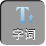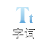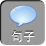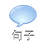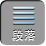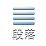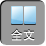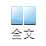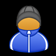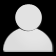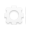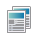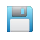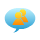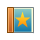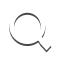-AA+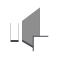1．数字黑洞 6174496

4321-1234= 3087

8730-0378= 8352

8532-2358= 6174

8832-2388= 1998

9981-1899= 7982

9872-2789= 7083

7830-0387= 7443

7443-3447= 3996

9963-3699= 6264

6642-2466= 4176

7641-1467= 6174

876-678= 198

981-189= 792

972-279= 693

963-369= 594

954-459= 495

2．两边对称的数——回文数

154+ 451= 605

605+ 506= 1111

1111就是一个回文数

1089+ 9801= 10890

10890+ 09801= 20691

20691+ 19602= 40293

40293+ 39204= 79497

79497又是一个回文数